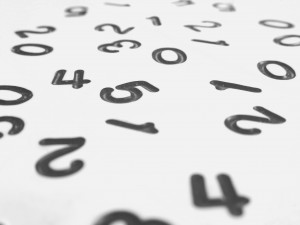# Scientific Inquiry & Reasoning Skills: General Mathematical Concepts and TechniquesYour knowledge of general mathematical concepts and techniques will be tested when you take the new MCAT exam. It is critically important that you are aware that questions regarding the natural, behavioral and social sciences sections will ask you to use specific mathematical concepts and techniques.

Some questions will ask you to demonstrate your knowledge of general mathematical concepts and techniques by doing the following:

• Interpret and Identify Linear, Semilog and Log-Log Scales
• Calculate Slopes Based on Data in Figures, Graphs and Tables
• Show a General Understanding of Significant Digits
• Display a General Understanding of the Use of Reasonable Numerical Estimates in Measurements and Calculations
• Demonstrate Your Ability to Use Metric Units, English Units and Dimensional Analysis
• Use Probability, Proportion, Ratio, Percentage and Square-Root Estimations to Perform Arithmetic Calculations
• Show a General Understanding of Exponentials and Logarithms, Scientific Notations and Simultaneous Equations
• Show a General Understanding of Trigonometric Concepts, Including Basic and Inverse Functions, Sin and Cos Values, and Relationships Between the Lengths of Sides of Right Triangles
• Show a General Understanding of Vector Addition and Subtraction as well as the Right-Hand Rule

It’s important to note that an understanding of calculus is not required for the MCAT exam. A periodic table of elements will also be provided to you as you take the exam.